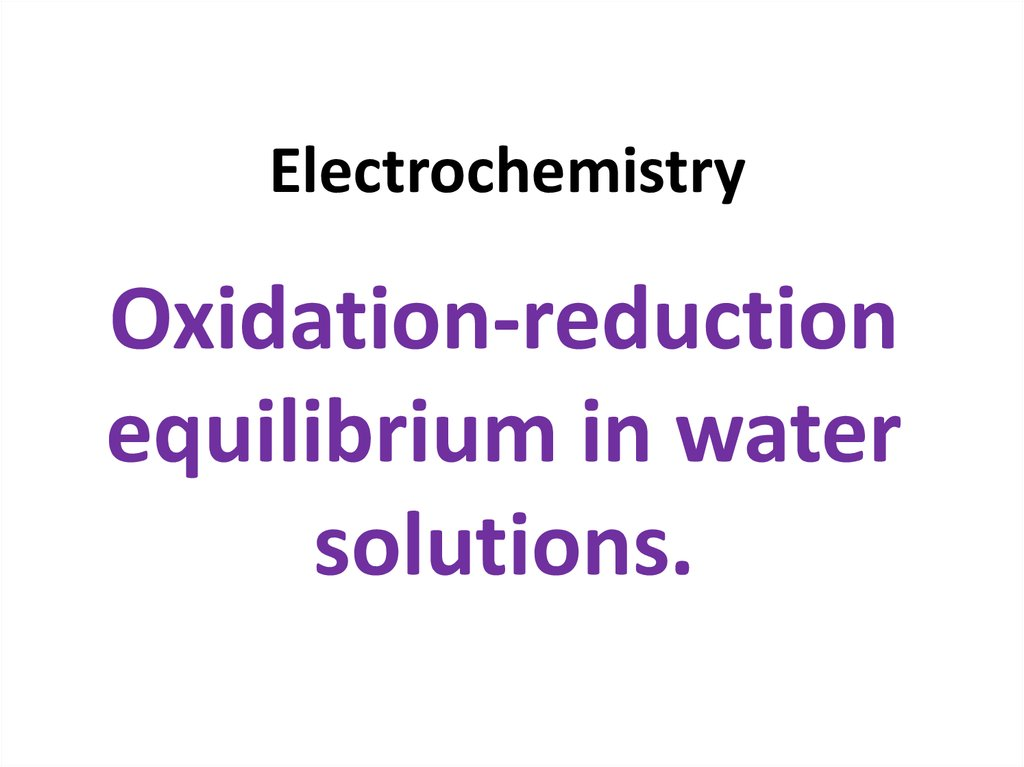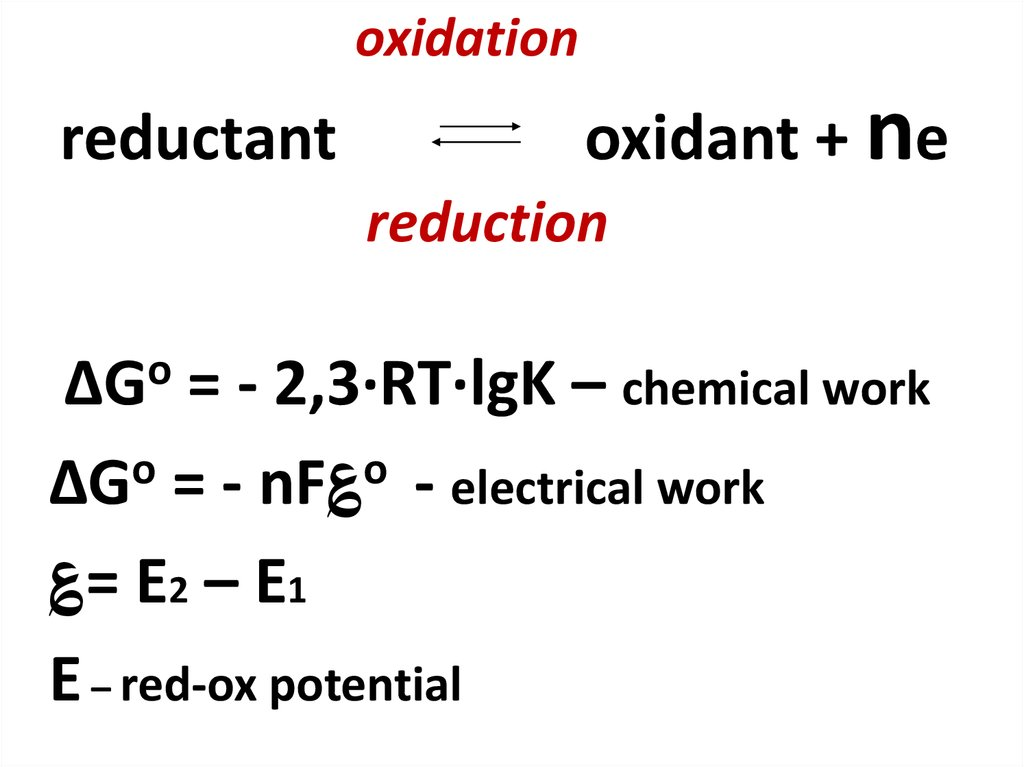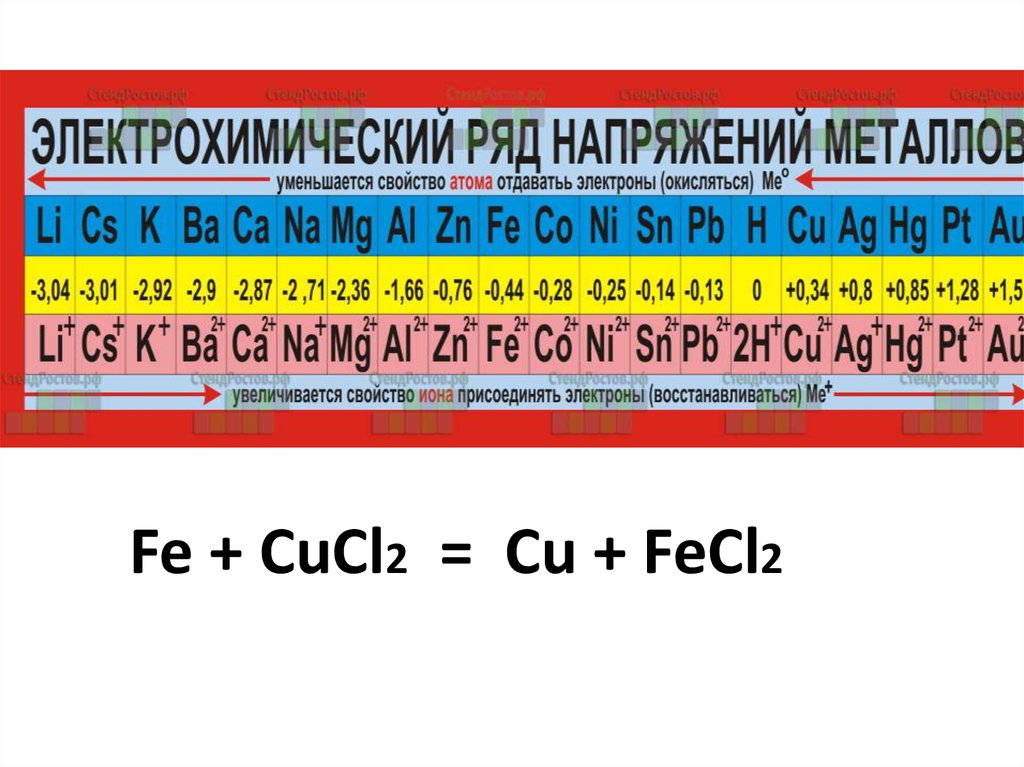# Electrochemistry. Oxidation-reduction equilibrium in water solutions

## 1. Electrochemistry

Oxidation-reduction
equilibrium in water
solutions.

## 2. oxidation reductant oxidant + ne reduction

o
ΔG
= - 2,3·RT·lgK – chemical work
ΔGo = - nF؏o - electrical work
؏= E2 – E1
E – red-ox potential

## 3.

Fe + CuCl2 = Cu + FeCl2

## 4. Criteria for spontaneous red-ox reactions

+7
+2
+2
+3
Mn + 5Fe
EoMn+7/Mn+2 = 1,51 В;
Mn + 5Fe
EoFe+3/Fe+2 = 0,77 В
EoMn+7/Mn+2 > EoFe+3/Fe+2
Mn+7 + 5ē → Mn+2
oxidant
Fe+2 - ē → Fe+3
reductant
1
n=5
5
ΔGo = -nF؏o
ΔGo < 0 for spontaneous reaction
؏o = 1,51 – 0,77 = 0,74 V
o = -5·96500·0,74 = -357050 J = -357,050 kJ.
ΔG
[email protected]

## 5. Red-ox potentials of biological systems

+
reductant
oxidant + 2e + 2 H
ΔGo = -2F؏o
Oxidant - acceptor of electrons and
protons

## 7. 1 type-electrodes

• Me Me+n – metal electrode
Me
Me+n + ne
• Pt(H2) H+ - hydrogen electrode
H2
+
2H +
2e

## 9. 2 type-electrodes

• Hg Hg2Cl2, KCl – calomel electrode
• Ag AgCl, KCl – silver chloride electrode
Ag
Ag+ + Cl-
In saturated KCl solutions
ESC = const.=0.202V
Ecal. = const. = 0.244V

3
Fe e Fe
2
Pt | Fe3+, Fe2+

## 12. Quinhydrone electrode Pt (QH) H+

Quinhydrone electrode
Pt (QH)
+
H
+ 2e + 2H+
quinone
hydroquinone

## 13. Nernst equation for electrode potential

ΔGo = - 2,3·RT·lgK – chemical work
ΔGo = - nF (E0 – E) - electrical work
Eox/red =
0
E ox/red +
(2,3RT/nF) lgK
2,3RT/F = 0.059 at T = 2890K, F= 96500, R=8.31
Eox/red = E0ox/red + (0.059/n) lg[ox]/[red]
E0ox/red – standard potential

## 14. Hydrogen electrode Pt (H2) | H+

½ Н2
Н+ + ē
Eox/red = E0ox/red + (0.059/n) lgK
EН2 = E0Н2 + (0.059/1) lg[H+]
E 0Н 2 = 0
Standard potential of hydrogen electrode
EН2 = - 0.059 pH

## 15. Nernst equation for biological redox system

reductant
oxidant + 2e + 2 H+
Eox/red =E0ox/red + (0.059/2) lg[ox][H+]2/[red]
Eox/red = E0ox/red – 0.059pH + 0.03 lg[ox]/[red]

## 16. Nernst equation for biological redox system at pH = 7

Eox/red = E0ox/red – 0.059pH + 0.03 lg[ox]/[red]
E0ox/red – 0.059pH = E0ox/red – 0.059 . 7

0
E ox/red = E0ox/red – 0.41
Standard potential of biological system at pH = 7

0
ox/red = E ox/red + 0.03 lg[ox]/[red]
E

## 17. Quinhydrone electrode Pt (QH) H+

Eox/red = E0ox/red – 0.059pH + 0.03 lg[ox]/[red]
EQH = E0 – 0.059pH + 0.03lg[quinone]/[hydroquinone]
QH
Quinhydrone = 1M quinone + 1Mhydroquinone
E
0
QH = E QH – 0.059pH

## 18. Galvanic (electrochemical) cell

- Zn | ZnSO4(р- р) || CuSO4(р- р) | Cu

## 19.

electromotive force (EMF)
؏= E2 – E1
؏о = ЕoCu – ЕoZn = 0,34 – ( - 0,76) = 1,1B
CuSO4 + Zn → ZnSO4 + Cu
reductant Zno - 2ē → Zn2+ oxidation
oxidant Cu2+ + 2ē → Cuo reduction
[email protected]

## 21. Potentiometry

Galvanic cell for pH measurements
(-) Pt | H 2 , H (pH ?) || KCl, AgCl | Ag ( )
Hydrogen electrode
EH
2
= -0.059 pH
Silver chloride
electrode
ESC =0.202V
ε измеренное E( ) E( ) E ХС E H 2
0,202 - (-0,059pH) 0,202 0,059pH
ε - E ХС ε - 0,202
pH
0,059
0,059

## 22.

1. Calculate the potential of quinhydronе
electrode in the 0.01M solution of HF. pKHF= 4,
E0QH= 0.699V
2. What is the degree of dissociation of a weak
acid, if the equilibrium potential of hydrogen
electrode immersed into the 0.2M solution of
this acid is equal to - 0.18V?
3. Estimate the value of ΔG0’ and determine
the direction of spontaneous reaction:

## 23.

4. Estimate the value of the equilibrium potential
of platinum electrode in the solution of two
salts: FeCl2 (0.25M) and FeCl3 (0.25M), E0Fe3+/Fe2+=
0.77V, t= 250C.
5. Determine the ratio of concentrations
[fumarate]/[succinate] if the potential of
platinum electrode in this solution is equal to
0.04V, t= 250C, E0’fum/suc= - 0.031V, pH = 7.
6. Diagram the cell consisting of the silver chloride
electrode in saturated KCl solution and hydrogen
electrode in the 0.01M solution of acetic acid .
What is the electromotive force (EMF) of this
cell? pKCH COOH = 4.75, ESC=0.202V